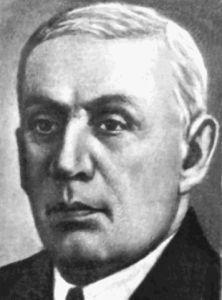## «Algebraic and geometric methods of analysis»

##### May 29 - June 1, 2023

dedicated to 160th anniversary of Dmytro Oleksandrovych Grave (25.08.1863 - 19.12.1939), academician of the Ukrainian Academy of Sciences, the first director of the Institute of Mathematics of NAS of Ukraine.Academician D. Grave is the author of a large number of results in many branches of theoretical and applied mathematics, in particular algebra, mathematical physics, mathematical problems of mechanics, and is even considered one of the pioneers of actuarial mathematics.

He made a significant contribution to the theory of Galois and the theory of Dedekind ideals, found some classes of algebraic equations of the fifth degree that are solvable in radicals, solved the problem of finding all integrals of the system of differential equations of the three-body problem independent of the law of action of forces, solved a number of problems of mathematical cartography. He is also the founder of a number of mathematical schools. Grave's students were such outstanding mathematicians as M. G. Chebotaryov, B. M. Delaunay, M. P. Kravchuk, O. Yu. Schmidt, A. M. Ostrovsky, Yu. D. Sokolov, N. I. Ahiezer, O. L. Naumov and many others.

In 2022 the National Academy of Sciences of Ukraine established the award named after D. O. Grave.

#### Conference topics

• Algebraic methods in geometry
• Differential geometry in the large
• Geometry and topology of differentiable manifolds
• General and algebraic topology
• Dynamical systems and their applications
• Geometric and topological methods in natural sciences
• Geometric problems in mathematical analysis

#### General information

Conference languages: Ukrainian and English.
Plenary (40 minutes) and section (20 minutes) presentations are planned.
Deadline for registration: May 20, 2023.
Deadline for sending abstracts: May 25, 2023.

The conference continues the traditional annual conference «Geometry in Odesa» holding from 2004 and was renamed to «Algebraic and geometric methods of analysis» in 2017. Here is the list of some previous conferences:
AGMA2017 - AGMA2018 - AGMA2019 - AGMA2020 - AGMA2021 - AGMA2022.

Developed by Sergiy Maksymenko - 2023